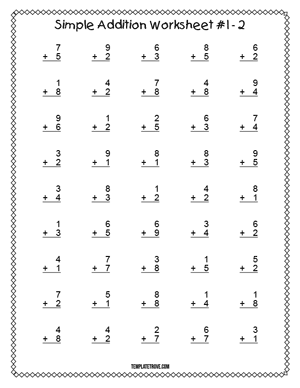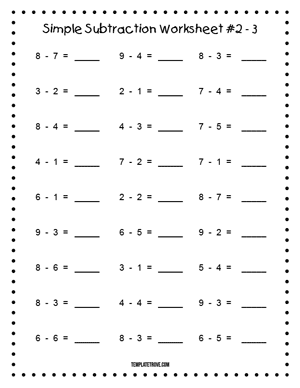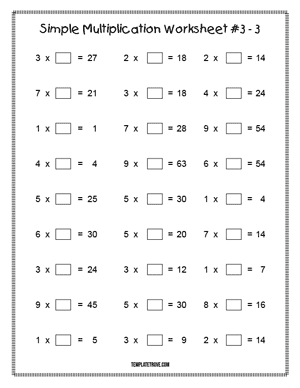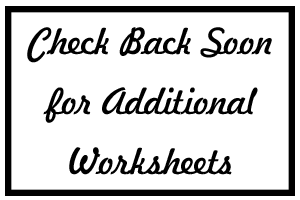﻿ Worksheets

# Worksheets

Free printable math worksheets with many more types of worksheets to be added as they are created. Template Trove's math worksheets are perfect for students and teachers.

All worksheets include answer keys if applicable and print on standard letter-size paper.

## Featured Worksheets

Math Simple Addition Worksheets - Printable simple addition math worksheets in five different styles.Math Simple Subtraction Worksheets - Five styles of basic math subtraction worksheets, perfect subtraction problem practice for those just learning subtraction.Math Simple Multiplication Worksheets - Five styles of basic math multiplication worksheets, perfect multiplication problem practice for those just learning how to multiply.Math Simple Division Worksheets - Printable simple division math worksheets in five different styles.